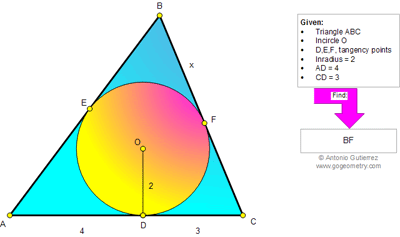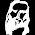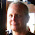## Tuesday, February 5, 2013

### Problem 852: Triangle, Incircle, Inradius, Tangent, Metric Relations

Geometry Problem
Level: Mathematics Education, High School, Honors Geometry, College.

Click the figure below to see the complete problem 852.1.Let BC=a, CA=b, AB=c, Area of ΔABC = S.

Then a=x+3, b=7, c=x+4, s=1/2(a+b+c)=x+7

S = √[12x(x+7)] = 2(x+7)
12x(x+7) = 4(x+7)^2
x = 7/2

2.half of angle A = Arctan(2/4)and half of angle B = arctan(2/3)
double these and subtract from 180 to find angle B. Then tan(B/2) = 2/x or
2/tan(B/2) = x = 3.5
Not as elegant as the solution above with more rounding involved. But fewer calculations.

3.Sorry, the first line should read:
half of angle A = Arctan(2/4)and half of angle C = arctan(2/3)